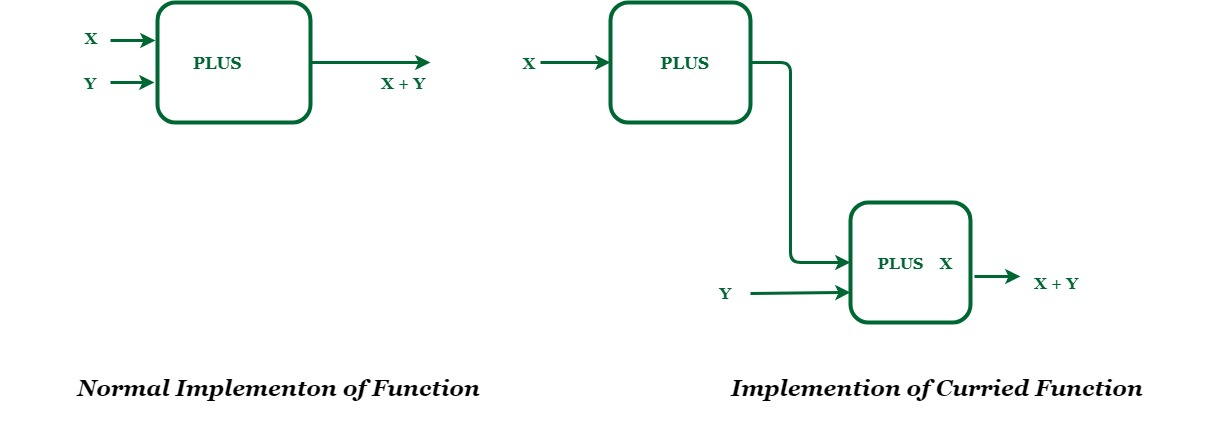Skip to content
Related Articles
Higher Order Functions and Currying
• Difficulty Level : Basic
• Last Updated : 27 Apr, 2018

Introduction:
Generally while programming, we use first class functions which means that the programming language treats functions as values – that you can assign a function into a variable, pass it around. These functions do not take other functions as parameters and never has any function as their return type.To overcome all these shortcomings of first-class functions, the concept of Higher Order function was introduced.

Why we need them?
In Imperative programming languages like (C/C++), we use functions very often or actually we can say that functions play a major role when programming in these languages. A typical function can be defined by function name, it’s return type and parameters it takes. We usually give int, char, pointer etc as parameter but is it possible to give one function as parameter to other function or can a function return another function as it’s result?

The answer is yes! The functions which take at least one function as parameter or returns a function as it results or performs both is called Higher Order Function. Many languages including- Javascript, Go, Haskell, Python, C++, C# etc, supports Higher Order Function.It is a great tool when it comes to functional programming.

Currying:
Functions can be classified on the basis of the number of inputs they accept like binary function will take two inputs while a unary function will take only a single input. In Haskell, every function takes only one input that is every function in Haskell can be said unary. Then it is not possible to implement a function which take multiple parameters? Of course, it is possible, by a methodology called currying (named after Haskell Curry, scientist who made this methodology popular and invented Haskell). In currying every function takes only one argument and returns a function. While the last function in this series will return the desired output.

Currying is the methodology of translating the evaluation of a function that takes multiple arguments into evaluating a sequence of functions, each with a single argument.

A Simple Example of Currying:
Let’s take an example, PLUS is a function which adds two number

1. We wish to add two numbers X and Y. X will be the input to PLUS function which returns a function named PLUS X.
2. PLUS X function takes one number and add X to it. Now input to this function will be Y. Final output will be X + Y.Advantages of Higher Order Functions:

By use of higher order function, we can solve many problems easily. For example we need to build a function which takes a list and another function as it’s input, applies that function to each element of that list and returns the new list. In Haskell, this could be done really easily using the inbuilt higher order function called map.Definition of map is:

```map :: (a -> b) -> [a] -> [b]
map _ [ ] = [ ]
map f (x : xs) = f x : map f xs  ```

Here,

• The first line is function initialisation.
• The :: symbol stands for “is of the type”.
• [a] represents a list of similar element, entity written after last -> is always the return type of the function.A function in Haskell always return only one entity.
• (a->b) defines a function from ‘a’ to ‘b’. We used recurrence to define map,
[] denotes empty list and _ denotes “anything”.
• Second line depicts that if empty list and any function is input then the output will be empty list.
• x : xs is used to take out elements one by one from list, x is the first element (head) and xs is remaining list (tail). : sign stands for concatenation. So in a nutshell, the third line is taking each element from the list and applying function ‘f’ on them and concatenating it with remaining list.

Example:

```map (+7) [1, 2, 3, 4, 5]
will return the list [8, 9, 10, 11, 12]. Here +7 is the function.```My Personal Notes arrow_drop_up